Jill

•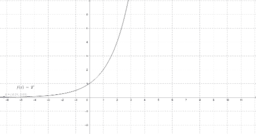Inverse Function Trace

Activity

Jill

•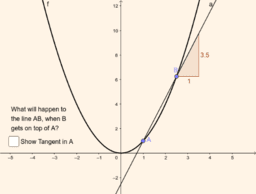Copy of Average Rate of Change vs Instantaneous rate of change

Activity

Jill

•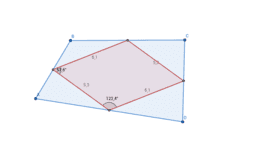Activity

Jill

•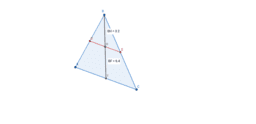What do you notice about the lengths of BF and BH?

Activity

Jill

•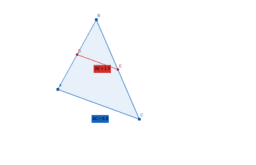What do you notice about the lengths of DE and AC?

Activity

Jill

•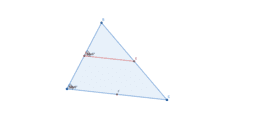Activity

Jill

•Triangle - Midpoints & Medians

Activity

Jill

•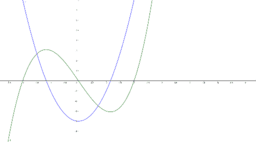Increasing and Decreasing

Activity

Jill

•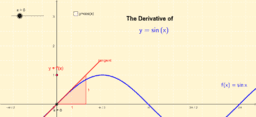Constructing the Graph of the Derivative of f(x)=sin(x)

Activity

Jill

•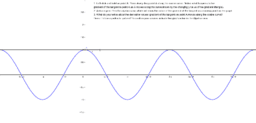Derivatives of sinx and cosx

Activity

Jill

•Derivative of cos(x)

Activity

Jill

•Constructing the Graph of the Derivative of f(x)=sin(x)

Activity

Jill

•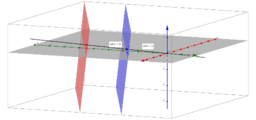Skew Lines

Activity

Jill

•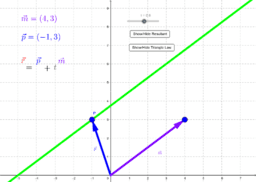Vector Equation of a Line in 2-Space

Activity

Jill

•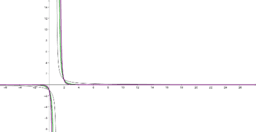Investigate B: Approaching positive or negative infinity?

Activity

Jill

•Investigate A: Graph of a Rational Function

Activity

Jill

•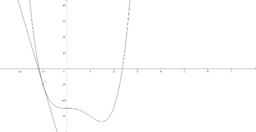Investigation - derivatives of a function

Activity

Jill

•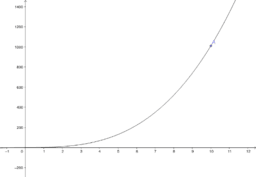Graph of f(x)=x^3+x, 0<=x<=10

Activity

Jill

•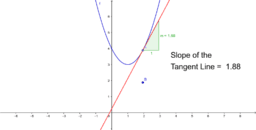Derivative of a Function as Slope of Tangent Line

Activity

Jill

•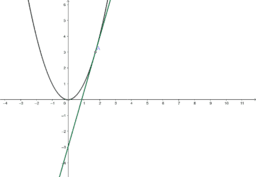Verifying instantaneous rate of change at a point

Activity

Jill

•Verifying instantaneous rate of change at a point

Activity

Jill

•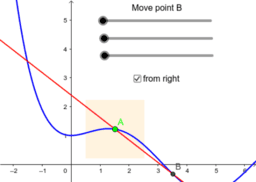Secant and Tangent

Activity

Jill

•Secant and Tangent

Activity

Jill

•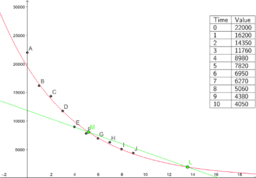Activity

Jill